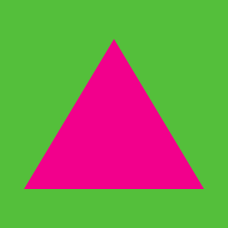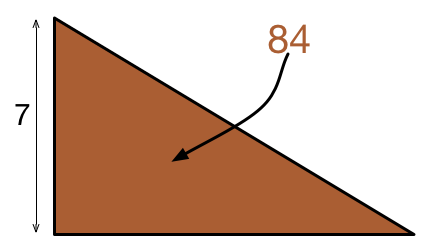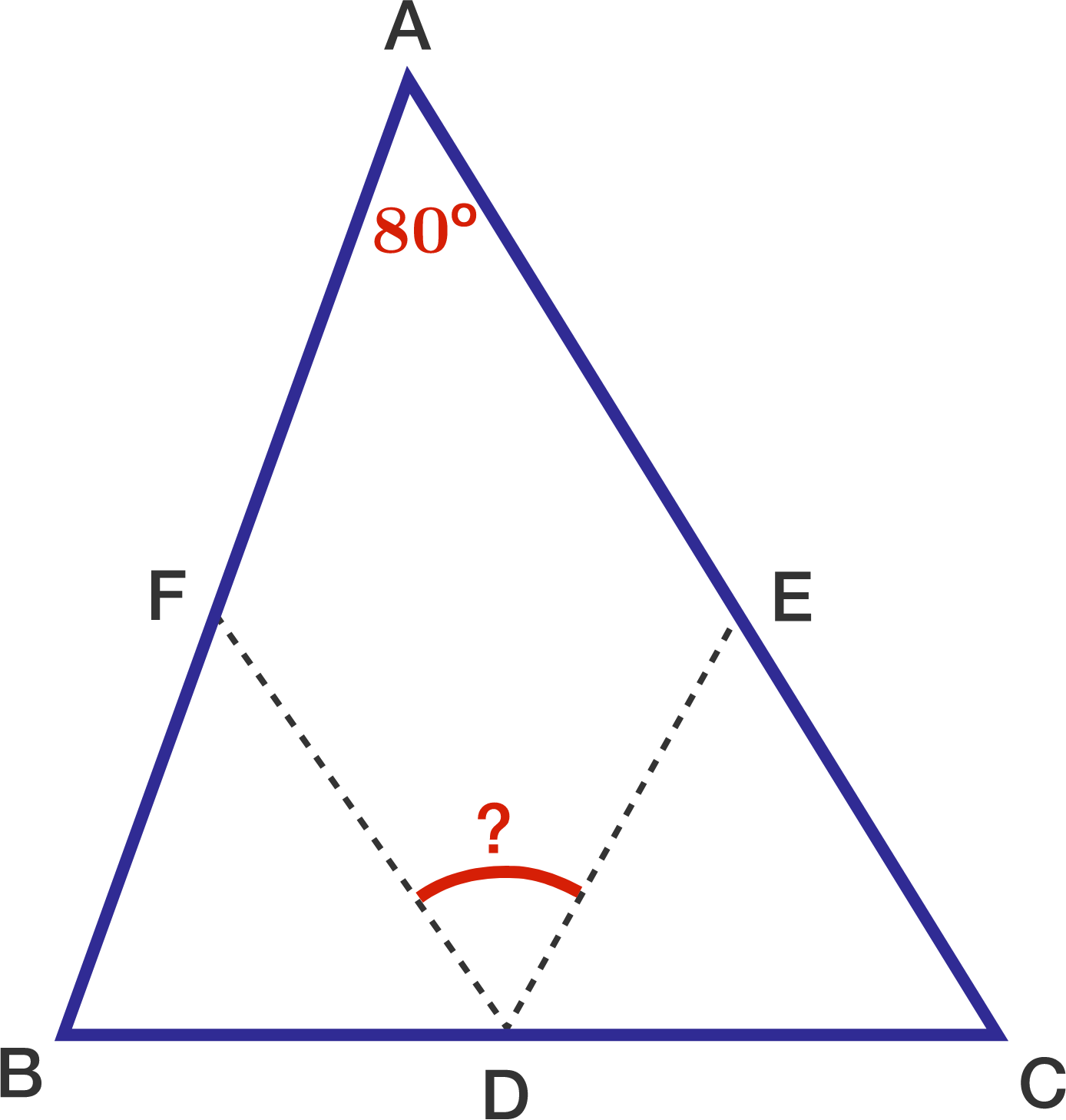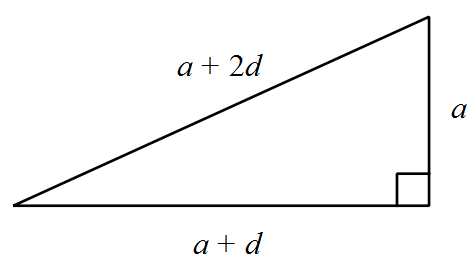Geometry

# Properties of Triangles: Level 1 Challenges

Triangle $ABC$ has the property that $\angle A+\angle B= \angle C$. Find the measure of $\angle C$ in degrees.A right triangle has a leg of length $7\text{ m}$ and an area of $84\text{ m}^2$. What is its perimeter?In $ABC$, sides $AB$ and $AC$ are equal in length and $\angle A$ measures $80^\circ$.

If the points D, E, and F are on sides BC, CA, and AB respectively, such that $CE= CD,BF=BD$, then what is $\angle EDF$?The sides of a right triangle are $a$, $a+d$ and $a+2d$ with $a$ and $d$ both positive. What is the value of $a:d$?

Note: Image drawn not up to scale.Arjit drew an isosceles right angled triangle on the board. What is the measure of the base angle?

×